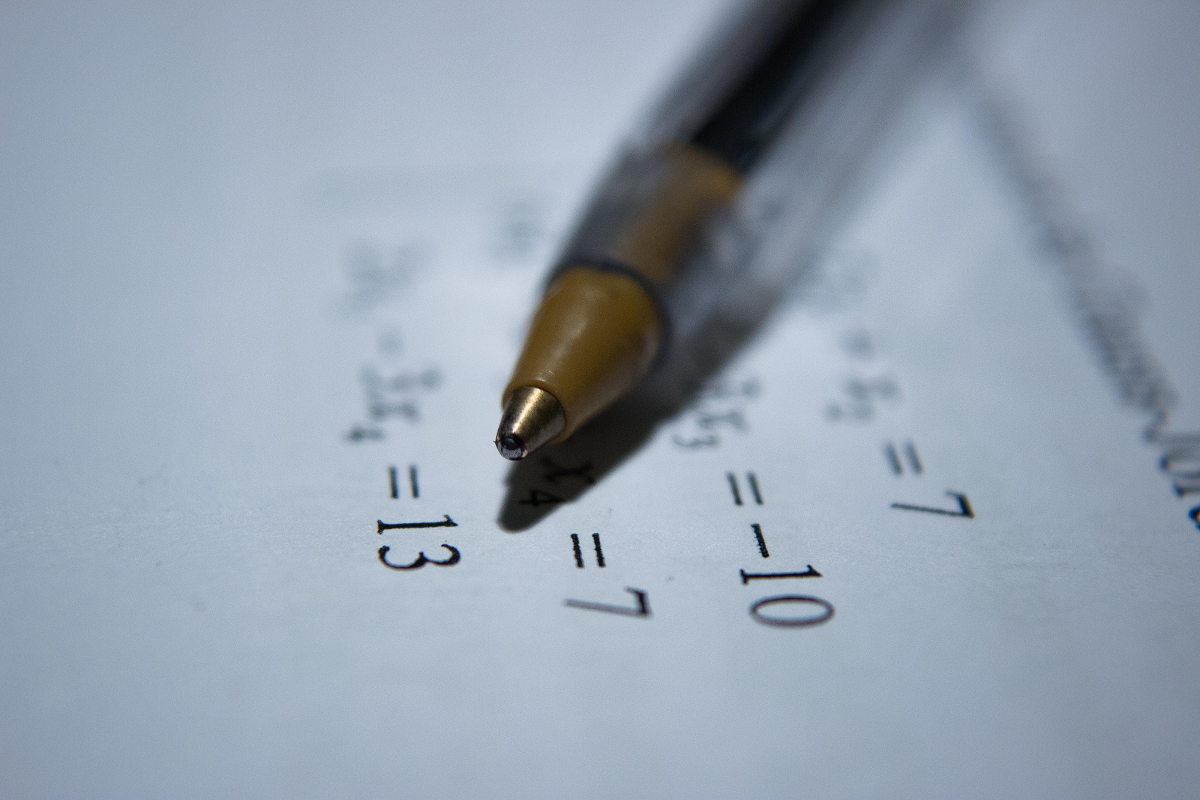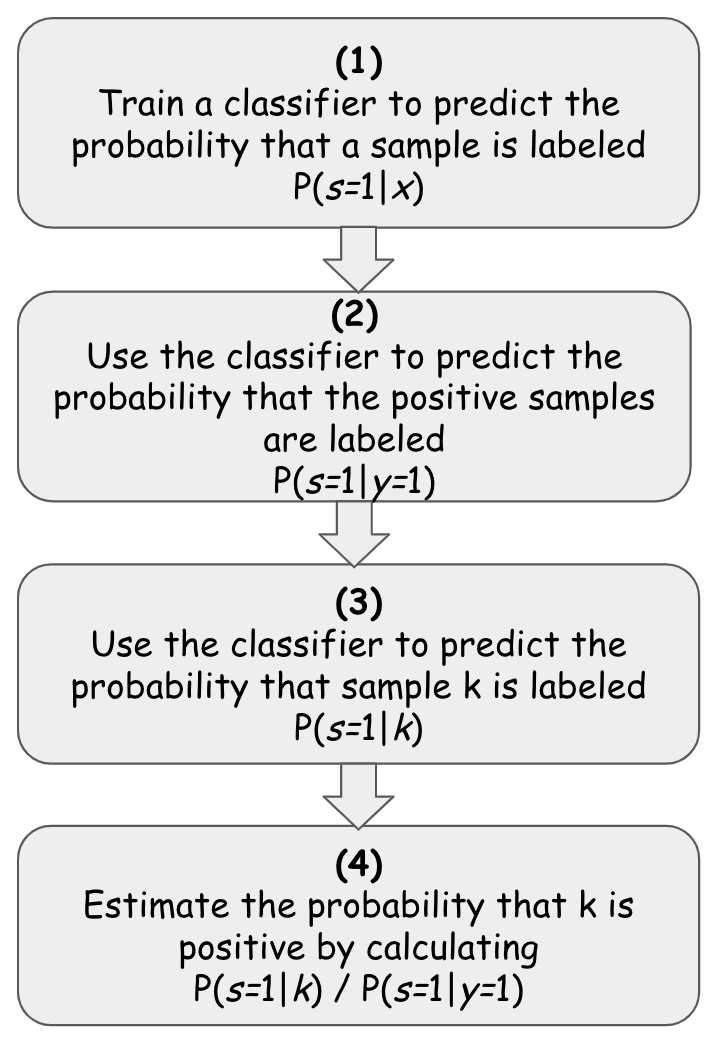# PU Learning简介:对无标签数据进行半监督分类

## 一点点理论E＆N方法本质上认为，在给定一个具有正样本和未标记样本的数据集的情况下，某个样本为正的概率[P（y = 1 | x）]等于一个样本被标记的概率[P（s = 1 | x）]除以一个正样本被标记的概率[P（s = 1 | y = 1）]。

（1）将分类器使用在包含标签和无标签样本的数据集上，同时使用已标记的指示器作为目标y,以这种方式拟合分类器对其进行训练，以预测给定样本x被标记的概率P（s = 1 | x）。
（2）使用分类器来预测数据集中已知正样本被标记的概率，使用预测的结果表示对正样本被标记的概率— P（s = 1 | y = 1 | x）

（3）使用我们训练的分类器（1）来估计K被标记的概率或者P(s=1|k)
（4）一旦我们估计了P（s = 1 | k），我们就可以通过将k除以在步骤（2）中估计的P（s = 1 | y = 1）来对k进行分类，然后获得它属于这两个类的实际概率。## 现在编写代码并进行测试

# prepare data
x_data = the training set
y_data = target var (1for the positives and not-1for the rest)
# fit the classifier and estimate P(s=1|y=1)
classifier, ps1y1 =
fit_PU_estimator(x_data, y_data, 0.2, Estimator())
# estimate the prob that x_data is labeled P(s=1|X)
predicted_s = classifier.predict_proba(x_data)
# estimate the actual probabilities that X is positive# by calculating P(s=1|X) / P(s=1|y=1)
predicted_y = estimated_s / ps1y1


fit_PU_estimator（）方法完成了2个主要任务：它适合您在正样本和未标记样本的数据集中选择合适的分类器，然后估计正样本被标记的概率。相应地，它返回一个拟合的分类器（已学会估计给定样本被标记的概率）和估计概率P（s = 1 | y = 1）。 之后，我们要做的就是找到P（s = 1 | x）或x被标记的概率。因为分类器被这样训练过，所以我们只需要调用其predict_proba（）方法即可。最后，为了对样本x进行实际分类，我们只需要将结果除以已经得到的P（s = 1 | y = 1）。

pu_estimator, probs1y1 = fit_PU_estimator(
x_train,
y_train,
0.2,
xgb.XGBClassifier())

predicted_s = pu_estimator.predict_proba(x_train)
predicted_s = predicted_s[:,1]
predicted_y = predicted_s / probs1y1


fit_PU_estimator()方法本身的实现是非常简单的

deffit_PU_estimator(X,y, hold_out_ratio, estimator):# The training set will be divided into a fitting-set that will be used # to fit the estimator in order to estimate P(s=1|X) and a held-out set of positive samples# that will be used to estimate P(s=1|y=1)# --------# find the indices of the positive/labeled elementsassert (type(y) == np.ndarray), "Must pass np.ndarray rather than list as y"
positives = np.where(y == 1.)
# hold_out_size = the *number* of positives/labeled samples # that we will use later to estimate P(s=1|y=1)
hold_out_size = int(np.ceil(len(positives) * hold_out_ratio))
np.random.shuffle(positives)
# hold_out = the *indices* of the positive elements # that we will later use  to estimate P(s=1|y=1)
hold_out = positives[:hold_out_size]
# the actual positive *elements* that we will keep aside
X_hold_out = X[hold_out]
# remove the held out elements from X and y
X = np.delete(X, hold_out,0)
y = np.delete(y, hold_out)
# We fit the estimator on the unlabeled samples + (part of the) positive and labeled ones.# In order to estimate P(s=1|X) or  what is the probablity that an element is *labeled*
estimator.fit(X, y)
# We then use the estimator for prediction of the positive held-out set # in order to estimate P(s=1|y=1)
hold_out_predictions = estimator.predict_proba(X_hold_out)
#take the probability that it is 1
hold_out_predictions = hold_out_predictions[:,1]
# save the mean probability
c = np.mean(hold_out_predictions)
return estimator, c

defpredict_PU_prob(X, estimator, prob_s1y1):
prob_pred = estimator.predict_proba(X)
prob_pred = prob_pred[:,1]
return prob_pred / prob_s1y1


===>> load data set <<===data size: (1372, 5)Target variable (fraud or not):
07621610===>> create baseline classification results <<===Classification results:f1: 99.57%
roc: 99.57%
recall: 99.15%
precision: 100.00%===>> classify on all the data set <<===Target variable (labeled or not):
-112191153Classification results:f1: 90.24%
roc: 91.11%
recall: 82.62%


## 几个要点

Deephub翻译组：gkkkkkk09-22116610-056071
05-041920
04-14964
03-255621
12-031349
03-25443
07-122377
04-20440
04-212259
04-235154
06-15607
05-311103
05-083403
10-25656
10-037099
09-201万+
12-2824